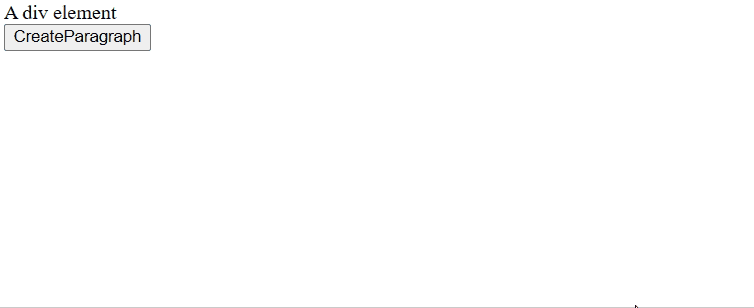# HTML DOM createElement() Method

In an HTML document, the document.createElement() is a method used to create the HTML element. The element specified using elementName is created or an unknown HTML element is created if the specified elementName is not recognized.

Syntax

`let element = document.createElement("elementName");`

In the above syntax, elementName is passed as a parameter. elementName specifies the type of the created element. The nodeName of the created element is initialized to the elementName value. The document.createElement() returns the newly created element.

Example 1: This example illustrates how to create a <p> element. Input :

## HTML

 `` `<``html``>` `<``head``>` `    ``<``script``>` `        ``function createparagraph() {` `            ``let x = document.createElement("p");` `            ``let t =` `                ``document.createTextNode("Paragraph is created.");` `            ``x.appendChild(t);` `            ``document.body.appendChild(x);` `        ``}` `    ``` ``   `<``body``>` `    ``<``button` `onclick``=``"createparagraph()"``>CreateParagraph` `` ``

Output:Explanation:

• Create a text node using document.createTextNode().
• Now, append the text to <p> using appendChild().
• Append the <p> to <body> using appendChild().

Example 2: This example illustrates how to create a <p> element and append it to a <div> element. Input :

## HTML

 `` `<``html``>` `<``head``>` `    ``<``script``>` `        ``function createparagraph() {` `            ``let x = document.createElement("p");` `            ``let t =` `                ``document.createTextNode("Paragraph is created.");` `            ``x.appendChild(t);` `            ``document.getElementById("divid").appendChild(x);` `        ``}` `    ``` `` `<``body``>` `    ``<``div` `id``=``"divid"``> A div element` `    ``<``button` `onclick``=``"createparagraph()"``>CreateParagraph` `` ``

Output:Supported Browser: The browsers supported by DOM createElement() Method are listed below: